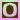Go to content
Two points are chosen at random in the interval [0, 1]; X denotes the first point chosen and Y denotes the second point chosen. Random variable U gives the type of triangle that can be formed from the three sub-intervals:
1. U = 0: the pieces do not form a triangle.
2. U = 1: the pieces form an obtuse triangle.
3. U = 2: the pieces form an acute triangle.
The first picture box shows the outcome of the experiment graphically. On each update, the cut points X and Y are shown as red dots, and the triangle is sketched when U = 1 or U = 2. The second picture box shows the sample space and the three events of interest:
1. U = 2 consists of the 2 interior regions.
2. U = 1 consists of the 6 middle regions.
3. U = 0 consists of the outer regions.
On each run, (X, Y) is shown as a red dot in the scatterplot, and is recorded in the first table on each update The probability density function of U is shown in blue in the graph box and is recorded in the graph table. On each update, the empirical density of U is shown in red in the graph box and is recorded in the graph table. Additionally, the value of U is recorded in the first table on each update.an applet from PSOL from UAH

## Glossary

### acute

An angle less than 90 degrees.

### cut

A set of edges whose removal separates a network into two parts, where one part contains the source and the other part contains the sink.

### density

the ratio of the mass of an object to its volume

### function

A rule that connects one value in one set with one and only one value in another set.

### graph

A diagram showing a relationship between two variables.
The diagram shows a vertical y axis and a horizontal x axis.

### obtuse

an angle between 90 and 180 degres

### random variable

a variable that represents the values obtained when measurements are taken from a population in the real world; it must take a numerical value.

### sample space

The list of all possible outcomes from an experiment.

### variable

A value which is unknown and free to vary, often denoted by x or y.

Full Glossary List

## This question appears in the following syllabi:

SyllabusModuleSectionTopicExam Year
AQA A-Level (UK - Pre-2017)S1ProbabilityExperimental-
AQA AS Maths 2017StatisticsProbabilityExperimental Probabilities-
AQA AS/A2 Maths 2017StatisticsProbabilityExperimental Probabilities-
CBSE XII (India)ProbabilityProbabilityRepeated independent (Bernoulli) trials and binomial distribution-
CBSE XI (India)Statistics and ProbabilityProbabilityRandom experiments, outcomes, sample spaces (set representation)-
CCEA A-Level (NI)S1ProbabilityExperimental-
CIE A-Level (UK)S1ProbabilityExperimental-
Edexcel A-Level (UK - Pre-2017)S1ProbabilityExperimental-
Edexcel AS Maths 2017StatisticsProbabilityExperimental Probabilities-
Edexcel AS/A2 Maths 2017StatisticsProbabilityExperimental Probabilities-
I.B. Higher Level5ProbabilityExperimental-
I.B. Standard Level5ProbabilityExperimental-
Methods (UK)M15ProbabilityExperimental-
I.B. (MSSL)3ProbabilityExperimental-
OCR A-Level (UK - Pre-2017)S1ProbabilityExperimental-
OCR AS Maths 2017StatisticsProbabilityExperimental Probabilities-
OCR MEI AS Maths 2017StatisticsProbabilityExperimental Probabilities-
OCR-MEI A-Level (UK - Pre-2017)S1ProbabilityExperimental-
Pre-Calculus (US)E4ProbabilityExperimental-
Pre-U A-Level (UK)ProbProbabilityExperimental-